# Iron Butterfly Payoff, Break-Even Points and R/R

This page explains iron butterfly payoff profile and the calculation of its maximum loss, maximum profit, break-even points and risk-reward ratio.

## Iron Butterfly Basic Characteristics

Iron butterfly is a non-directional short volatility strategy, typically used when a trader expects the underlying price to move sideways or stay at approximately the same level.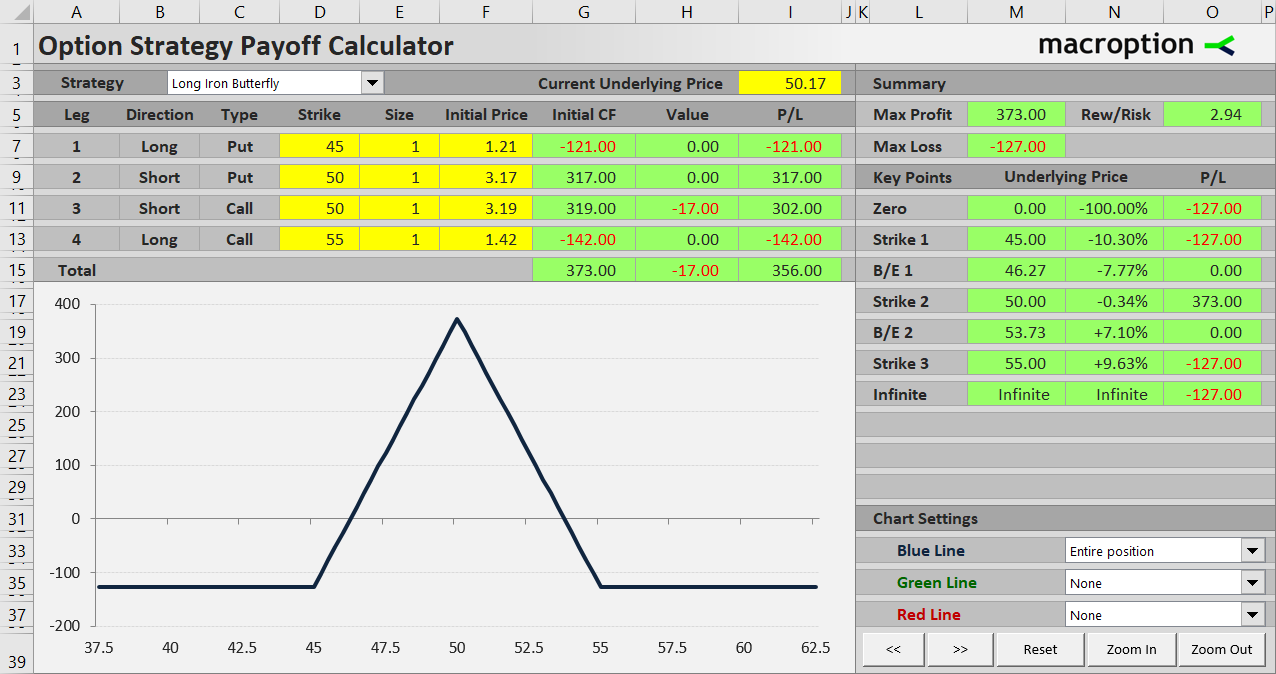The position consists of four different options with three different strikes:

When you look at the list above, you can see that the middle item (short put + short call with same strike) is actually a short straddle. The two remaining options (lower strike long put + higher strike long call) are a long strangle.

Iron butterfly is like a short straddle hedged by a long strangle. It has limited maximum profit (just like a short straddle) and limited risk (unlike a short straddle, thanks to the long put and long call).

We will use an example to explore the profit or loss under different scenarios and calculate maximum loss, maximum profit, break-even points and risk-reward ratio.

## Iron Butterfly Example

Let's create an iron butterfly with the following four transactions:

• Buy a \$45 strike put option for \$1.21 per share.
• Sell a \$50 strike put for \$3.17 per share.
• Sell a \$50 strike call (same strike as the put above) for \$3.19 per share.
• Buy a \$55 strike call for \$1.42 per share.

Typically, the middle strike will be the strike closest to the current underlying price and the distances between strikes will be equal (in our case both are \$5). This ensures the position has as little directional bias as possible.

## Initial Cash Flow

Iron butterfly is a credit strategy, which means cash flow is positive when opening the position. This is because the middle strike options being sold are typically more expensive than the lower strike put and the higher strike call being bought.

In our example, assuming position size of one contract (which represents 100 shares), initial cash flow equals \$317 plus \$319 received for the middle strike options minus \$121 paid for the lower strike put minus \$142 paid for the higher strike call = \$373 net premium received.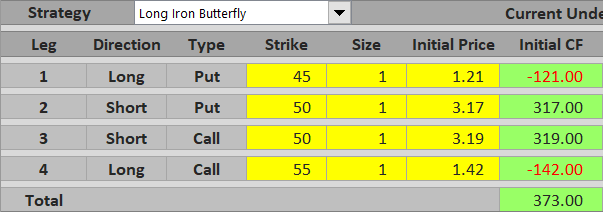## Maximum Profit

The net premium received at the beginning is actually the most you can make on this trade. As a short volatility strategy, iron butterfly makes most money when underlying price stays exactly at the middle strike. Above the middle strike, the short call gets in the money. Below the middle strike, the short put gets in the money.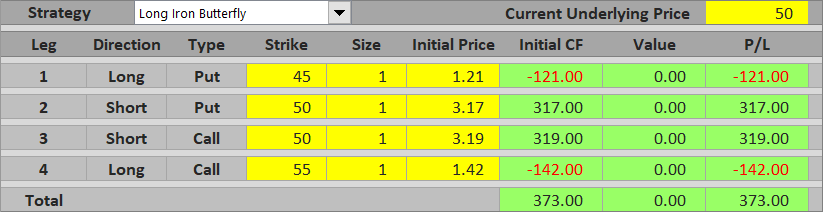Maximum profit from an iron butterfly equals net premium received and applies only when underlying price is exactly at the middle strike at expiration.

## Underlying above Middle Strike

Between the middle strike and the upper strike (\$50 and \$55 in our example), only one of the legs is driving P/L – the short call. All the other options are out of the money. The higher underlying price gets above the middle strike, the higher the short call value and the lower total profit.

For example, with underlying price at \$52, the short call option's value is \$52 – \$50 = \$2 per share = \$200 for one contract. Because we are short this option, its value contributes negatively to our P/L. All the other options are worth zero. Combined with initial cash flow, total P/L under this scenario is \$373 – \$200 = \$173 profit.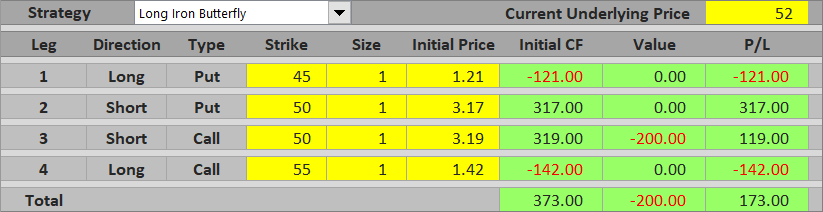If the underlying gets higher, at some point the short call option's value will exceed initial cash flow and the trade turns into a loss. For example, with underlying at \$54, the short call's value is \$400 and total P/L is – \$27, a small loss.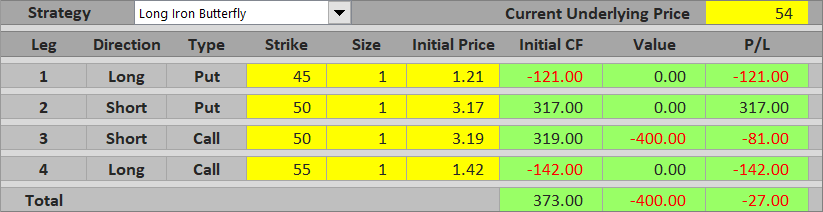Above the higher strike (\$55), the higher strike long call gets in the money and starts to limit the losses from the middle strike short call. Because both the calls have the same position size, further increase in the short call option's value will match further increase in the long call option's value.

As a result, P/L above the higher strike is constant and equal to net premium received minus the difference between the call strikes. This is the maximum possible loss from an iron butterfly trade. In our example it equals \$373 – \$500 = – \$127.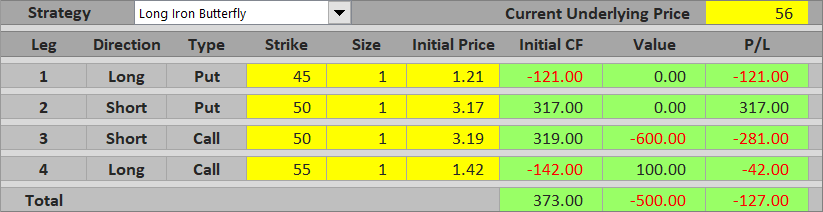The payoff profile above the middle strike is similar to bear call spread payoff. We have mentioned in the beginning that iron butterfly can be considered a combination of short straddle and long strangle. Alternatively, it can also be considered a combination of a bull put spread (long put + short put) and a bear call spread (short call + long call). Above the middle strike, both puts are out of the money and have no effect. The rest of the iron butterfly (short call + long call) is just like a bear call spread.

## Underlying below Middle Strike

Bellow the middle strike it works in the same way, only this time it is the put options driving total P/L and the calls are out of the money. In other words, it works just like a bull put spread.

Near the middle strike the short put's value is still smaller than net premium received and total P/L is a profit.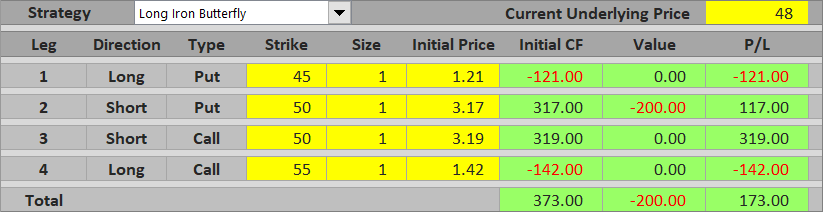Near the lower strike the trade turns into a loss, as the short put's value exceeds net premium received.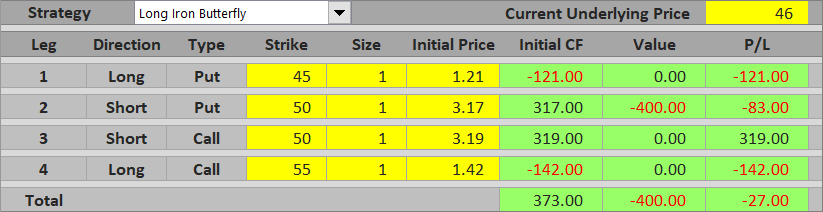Below the lower strike, the long put starts to offset further increase in the short put's value. Total P/L is constant and equal to maximum loss.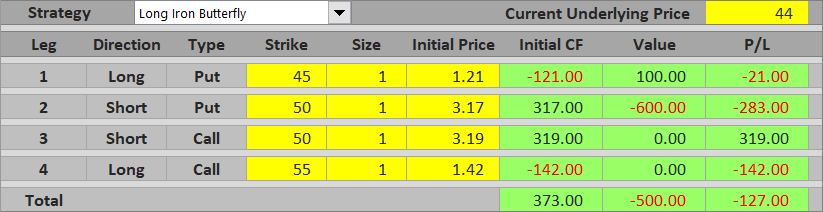## Iron Butterfly Break-Even Points

Iron butterfly strategy has two break-even points and, obviously, they can be found between the strikes.

The first break-even point is situated between the lower strike and the middle strike. It is the underlying price where the short put option's value matches net premium received.

The other break-even point, situated between the middle strike and the upper strike, is where the short call option's value equals net premium received.

Both break-even points have the same distance from the middle strike, equal to net premium received.

In our example the break-evens are:

B/E #1 = \$50 – \$3.73 = \$46.27

B/E #2 = \$50 + \$3.73 = \$53.73

## Iron Butterfly Risk-Reward Ratio

As you can see (from the payoff diagram as well as from the break-even point calculations above), the profit window for iron butterfly tends to be considerably narrower than the profit window of other short volatility strategies, like short straddle or iron condor. On the other hand, iron butterfly often has a very favorable risk-reward ratio. Of course, exact numbers for a particular trade depend mainly on strike selection and option prices.

We already know from the scenarios discussed above what the maximum loss (risk) and maximum profit (reward) are.

Maximum loss (risk) = higher strike – middle strike – net premium received

In our example:

Maximum profit = \$3.73 per share = \$373 per contract

Maximum loss = \$55 – \$50 – \$3.73 = \$1.27 per share = \$127 per contract

Using these figures, the risk-reward ratio is 1 : 373/127 or 1 : 2.94. In other words, potential profit is almost 3x greater than risk in this particular case.

## Iron Butterfly Payoff Diagram & Summary

Let's conclude with a payoff diagram and a summary.Below the lower (\$45) strike, the short put's effect is hedged by the long put and total P/L is constant, equal to maximum loss.

Between the lower (\$45) and the middle (\$50) strike, total P/L increases proportionally to underlying price. Break-even point is at middle strike – net premium received (\$46.27)

Maximum profit is only when underlying price ends up exactly at the middle strike (\$50) at expiration. It equals net premium received.

Between the middle (\$50) and upper (\$55) strike, total P/L decreases as underlying price rises. Break-even point is at middle strike + net premium received (\$53.73)

Above the upper strike (\$55), the long call starts to offset further increase in the short call value. Total P/L is constant and equal to maximum loss.

We already know that iron butterfly is a combination of short straddle and long strangle, or a combination of bull put spread and bear call spread.

A strategy often considered as an alternative to iron butterfly is iron condor, which unlike iron butterfly has different strikes for the short put and short call. This (other things being equal) results in wider distance between break-evens and higher probability of profit, but also in lower net premium received (or maximum profit), higher maximum loss and weaker risk-reward ratio.

By remaining on this website or using its content, you confirm that you have read and agree with the Terms of Use Agreement.

We are not liable for any damages resulting from using this website. Any information may be inaccurate or incomplete. See full Limitation of Liability.

Content may include affiliate links, which means we may earn commission if you buy on the linked website. See full Affiliate and Referral Disclosure.

We use cookies and similar technology to improve user experience and analyze traffic. See full Cookie Policy.